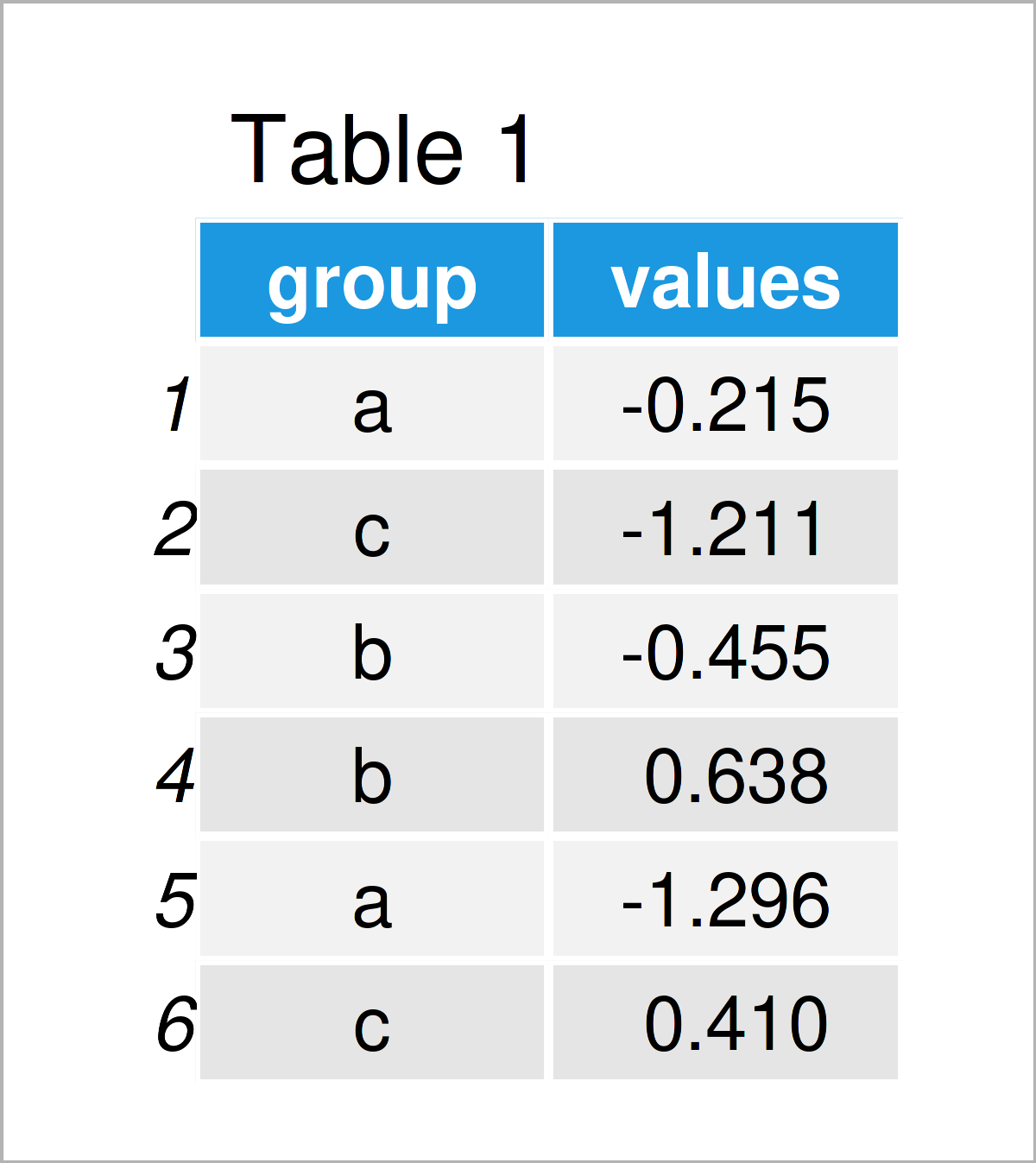# Add Number of Observations by Group to ggplot2 Boxplot in R (Example)

In this R tutorial you’ll learn how to add the count by group to a boxplot.

Let’s dive into it…

## Example Data, Software Packages & Basic Graph

We’ll use the following data as basement for this R tutorial.

```set.seed(395678)                             # Create example data
data <- data.frame(group = sample(letters[1:4], 100, replace = TRUE),
values = rnorm(100))Have a look at the table that got returned after executing the previous code. It shows the upper rows of the example data, and that the data consists of two variables called “group” and “values”. The variable group has the character class and the variable values is numerical.

To be able to use the functions of the ggplot2 package, we also need to install and load ggplot2:

```install.packages("ggplot2")                  # Install ggplot2 package

Next, we can plot our data:

```ggp <- ggplot(data, aes(group, values)) +    # Create default ggplot2 boxplot
geom_boxplot()
ggp                                          # Draw default ggplot2 boxplot```As shown in Figure 1, we have drawn a ggplot2 boxplot with the previous R programming syntax.

This boxplot does not show any count labels for the different groups yet. Let’s do this!

## Example: Add Sample Size by Group to ggplot2 Boxplot Using annotate() Function

This example demonstrates how to annotate the number of observations per group as text labels to each box of a ggplot2 boxplot.

To do this, we can apply the annotate function as shown below:

```ggp +                                        # Add counts by group to boxplot
annotate("text",
x = 1:length(table(data\$group)),
y = aggregate(values ~ group, data, median)[ , 2],
label = table(data\$group),
col = "red",
vjust = - 1)```By executing the previous R programming code we have managed to create Figure 2, i.e. a ggplot2 boxplot with sample sizes by group.

## Video & Further Resources

In case you need more information on the R programming codes of this tutorial, you may want to have a look at the following video on my YouTube channel. I’m explaining the content of this article in the video.

In addition, you may have a look at some of the other articles on this homepage:

At this point you should know how to add the observations by group to a boxplot in R programming. Don’t hesitate to let me know in the comments, if you have additional questions.

Subscribe to the Statistics Globe Newsletter

•